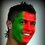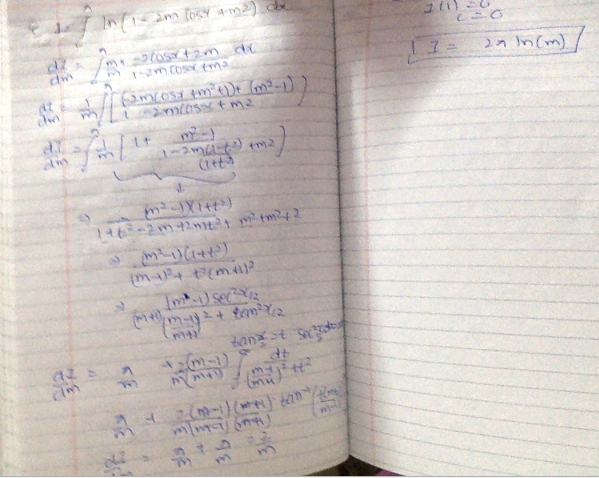# Integration

Let $\displaystyle I(m) = \int_0^\pi \ln (1 - 2m \cos x + m^2) \, dx$. Find the values of $\dfrac{I(9)}{I(3)}$ and $I(81)$.Note by Pratik Roy
4 years, 11 months ago

This discussion board is a place to discuss our Daily Challenges and the math and science related to those challenges. Explanations are more than just a solution — they should explain the steps and thinking strategies that you used to obtain the solution. Comments should further the discussion of math and science.

When posting on Brilliant:

• Use the emojis to react to an explanation, whether you're congratulating a job well done , or just really confused .
• Ask specific questions about the challenge or the steps in somebody's explanation. Well-posed questions can add a lot to the discussion, but posting "I don't understand!" doesn't help anyone.
• Try to contribute something new to the discussion, whether it is an extension, generalization or other idea related to the challenge.

MarkdownAppears as
*italics* or _italics_ italics
**bold** or __bold__ bold
- bulleted- list
• bulleted
• list
1. numbered2. list
1. numbered
2. list
Note: you must add a full line of space before and after lists for them to show up correctly
paragraph 1paragraph 2

paragraph 1

paragraph 2

[example link](https://brilliant.org)example link
> This is a quote
This is a quote
    # I indented these lines
# 4 spaces, and now they show
# up as a code block.

print "hello world"
# I indented these lines
# 4 spaces, and now they show
# up as a code block.

print "hello world"
MathAppears as
Remember to wrap math in $$ ... $$ or $ ... $ to ensure proper formatting.
2 \times 3 $2 \times 3$
2^{34} $2^{34}$
a_{i-1} $a_{i-1}$
\frac{2}{3} $\frac{2}{3}$
\sqrt{2} $\sqrt{2}$
\sum_{i=1}^3 $\sum_{i=1}^3$
\sin \theta $\sin \theta$
\boxed{123} $\boxed{123}$

Sort by:

Prateek refer to amit m agarwal u will get lot stuff like this

- 4 years, 10 months ago

thanks

- 4 years, 10 months ago

- 4 years, 11 months ago- 4 years, 10 months ago

Hope it helps.

P.S. this is an ai^2ts question . are you too in fiitjee ?

- 4 years, 10 months ago

yes

- 4 years, 10 months ago

Nilesh are u a faculty member there

- 4 years, 10 months ago

no i am just an average class 12 student.

- 4 years, 10 months ago

this solution is a great help thanks a lot man... do you have any other problems on integration like this . if you have then please do post.

- 4 years, 10 months ago

I m in fitjee bro ...

- 4 years, 10 months ago

1. Let f(x) = ax2 + bx + c where a,b,c are real numbers. Suppose f(−1),f(0), f(1) ∈ [−1,1]. Prove that |f(x)| ≤ 3/2 for all x ∈ [−1,1].pratik could u please give solution for this

- 4 years, 2 months ago